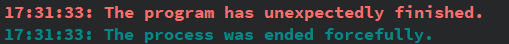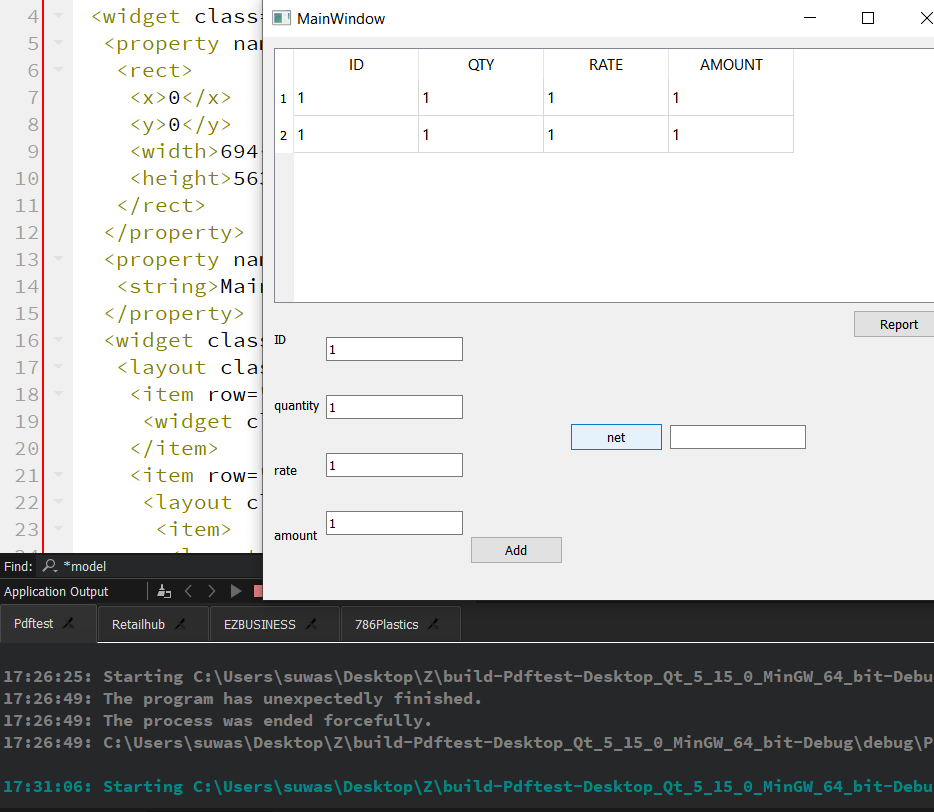# How to read data from a column of QTableWidget ?

• I have written this code to read data from table containing 4 column (4th being amount). I have error when reading data from QTableWidget, table name(table). After pressing net Pushbutton, program crashes.

void MainWindow::on_net_clicked()
{
int total=0;
int row=ui->table->rowCount();

``````for(int i=1;i<=row;i++){
QTableWidgetItem* a=ui->table->item(i,4);
int b=a->text().toInt();
total = (total + b);
}
QString gross= QString::number(total);
ui->le_gross->setText(gross);
``````

}• `ui->table->item(i,4)`

Where do you think that `4` point to? I only see 4 columns in your screenshot, not 5....

``````    QTableWidgetItem* a=ui->table->item(i,4);
int b=a->text().toInt();
``````

It "crashes" (on `a->text()`) because you do not test whether `a == nullptr`, which it will do, and you should always test for....

• @JonB
I will try what are you suggesting too. I have tried this method and looks like it is working. Is it proper?
//I didn't know column starts at 0; thought it start with 1 :""

void MainWindow::on_net_clicked()
{
int total=0;
//int row=ui->table->rowCount();

``````for(int i=0;i<ui->table->rowCount();i++){
int a=ui->table->item(i,3)->text().toInt();
total=total+a;
}
QString gross= QString::number(total);
ui->le_gross->setText(gross);
``````

}

• //I didn't know column starts at 0; thought it start with 1 :""

We are in C/C++ realm, not Pascal/Visual Basic :)

Your code will now work. Provided you have >= 4 columns.